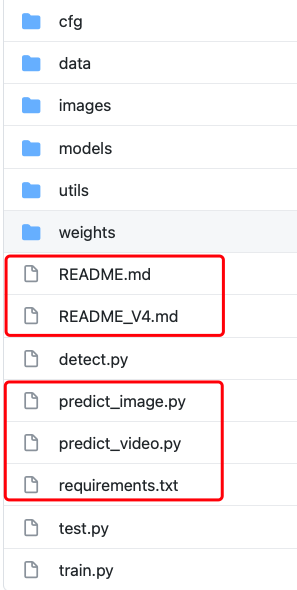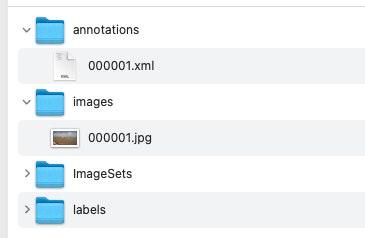﻿• 价格透明
• 信息保密
• 进度掌控
• 售后无忧# YOLO-V4 Pytorch版本训练自建数据集和预测

## 1. 程序下载

``````git clone https://github.com/hx-0614/yolov4-pytorch-learing.git
``````## 2. 代码结构解析

``````|cfg  # 存储模型结构配置文件的地方。
|------|yolov4.cfg
|------|......
|data  # 数据读取文件参数。
|------|hyp.scratch.yaml  # 训练相关的参数，我一般使用默认。
|------|coco.data  # 训练集，验证集，数据集类别，数据集标签类别参数文件。
|------|coco.names  # 数据集标签类别。这个文件一般测试的时候使用，貌似train没用到。
|------|......
|images  # 存储模型结构的图片。
|------|......
|models  # 构建模型的脚本文件。
|------|......
|utils  # 程序执行过程中要用到的其他相关函数的脚本文件。
|------|......
|weights  # 存放模型的文件，我是把预先训练模型存到了这里。
|------|......

|detect.py  # 模型自带的批量推理代码，默认是输入一个图片文件夹，然后输出检测带有框的文件夹。
|test.py  # 测试mAP的脚本文件。其中train.py 也会调用它，也可以单独使用，主要是针对coco.data里面的测试集路径进行测试。
|train.py  # 训练脚本文件。

### 我新建的一些脚本 ###
|predict_image.py  # 预测一张图片的脚本。
|predict_video.py  # 预测一段视频的脚本。
|run_train.sh  # 运行train.py的shell脚本。

|requirements.txt  # 需要的相关环境下载文件。

``````

## 3. 训练自己的数据集

### 3.1. 首先需要准备自己的数据集``````# makeTxt.py 制作训练集和测试集列表的脚本。
# 需要将脚本中的所有路径进行更改，手动改就好，改成自己数据集存储的地方，我建议大家使用绝对路径（不要出现中文）。
import os
import random

trainval_percent = 0.2  # 可自行进行调节
train_percent = 1
xmlfilepath = '../annotations'
txtsavepath = '../images'
total_xml = os.listdir(xmlfilepath)

num = len(total_xml)
list = range(num)
tv = int(num * trainval_percent)
tr = int(tv * train_percent)
trainval = random.sample(list, tv)
train = random.sample(trainval, tr)

# ftrainval = open('ImageSets/Main/trainval.txt', 'w')
ftest = open('../ImageSets/test.txt', 'w')
ftrain = open('../ImageSets/train.txt', 'w')
# fval = open('ImageSets/Main/val.txt', 'w')

for i in list:
name = total_xml[i][:-4] + '\n'
if i in trainval:
# ftrainval.write(name)
if i in train:
ftest.write(name)
# else:
# fval.write(name)
else:
ftrain.write(name)

# ftrainval.close()
ftrain.close()
# fval.close()
ftest.close()
``````
``````#!/usr/bin/env python
# -*- coding:utf-8 -*-
# voc_label.py 生成YOLO可以读取的数据集格式，最后会在labels文件夹下面生成每个图片的标签文件，txt结尾。
import xml.etree.ElementTree as ET
import pickle
import os
from os import listdir, getcwd
from os.path import join

sets = ['train', 'test']

classes = ['a', 'b', 'c']  # 自己训练的类别

def convert(size, box):
dw = 1. / size
dh = 1. / size
x = (box + box) / 2.0
y = (box + box) / 2.0
w = box - box
h = box - box
x = x * dw
w = w * dw
y = y * dh
h = h * dh
return (x, y, w, h)

def convert_annotation(image_id):
in_file = open('../annotations/%s.xml' % (image_id))
out_file = open('../labels/%s.txt' % (image_id), 'w')
tree = ET.parse(in_file)
root = tree.getroot()
size = root.find('size')
w = int(size.find('width').text)
h = int(size.find('height').text)

for obj in root.iter('object'):
difficult = obj.find('difficult').text
cls = obj.find('name').text
if cls not in classes or int(difficult) == 1:
continue
cls_id = classes.index(cls)
xmlbox = obj.find('bndbox')
b = (float(xmlbox.find('xmin').text), float(xmlbox.find('xmax').text), float(xmlbox.find('ymin').text),
float(xmlbox.find('ymax').text))
print(root.find('filename').text)
bb = convert((w, h), b)
out_file.write(str(cls_id) + " " + " ".join([str(a) for a in bb]) + '\n')

wd = getcwd()
for image_set in sets:
if not os.path.exists('../labels/'):
os.makedirs('../labels/')
list_file = open('../%s.txt' % (image_set), 'w')
for image_id in image_ids:
list_file.write('../images/%s.jpg\n' % (image_id))
convert_annotation(image_id)
list_file.close()
``````

### 3.2. 修改相关的配置文件

（1）修改data文件夹下面的配置文件。主要修改两个文件，也可以新建两个文件一个是 .data结尾一个是 .names结尾的文件，如下：

``````# my.data
# train和test的路径根据自己的生成情况更改，但是train.txt里面存储的是路径。不是图片的名字。
train: ../train.txt
val: ../test.txt
test: ../test.txt

# number of classes
nc: 3

# class names
names: ['a', 'b', 'c']
``````
``````# my.names
# 这个文件存储类别，写的时候把这两行备注去掉以防出bug。
a
b
c
``````

（2）修改cfg文件，模型结构文件，以yolov4.cfg为例子。

（3）预先训练模型。这里把预先训练模型上传到了百度网盘，大家可以下载。

### 3.3. 执行训练代码train.py

``````# 训练脚本shell文件
python train.py --weights weights/yolov4.weights --cfg  cfg/yolov4.cfg --epochs 100 --batch-size 4 --img-size 512  --data data/xinye.yaml --device 0 --logdir runs/
``````
``````pip install -r requirements.txt -i https://pypi.tuna.tsinghua.edu.cn/simple
bash run_train.sh
``````

``````class Mish(nn.Module):
def __init__(self):
super().__init__()
def forward(self,x):
x = x * (torch.tanh(F.softplus(x)))
return x
``````

## 4. 预测图片和视频

### 4.1. predict_image.py

``````if __name__ == "__main__":
"""
letterbox() 图片resize后空白区域填充函数
non_max_suppression() nms
scale_coords_() 图片尺寸还原

init() 初始化模型
process() 主运行函数。输入参数为model，img 返回每张图得到的bbox结果 json格式
"""
model = init()
res = process(model, img, img0)
``````

# 其他相关连接

YOLO-V1:https://blog.csdn.net/worewolf/article/details/116563934?spm=1001.2014.3001.5501
YOLO-V2:https://blog.csdn.net/worewolf/article/details/116569183?spm=1001.2014.3001.5501
YOLO-V4:https://blog.csdn.net/worewolf/article/details/116569208?spm=1001.2014.3001.5501
github：https://github.com/hx-0614/yolov4-pytorch-learing

# 后续### 低价透明### 金牌服务### 信息保密